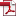• KSII Transactions on Internet and Information Systems
Monthly Online Journal (eISSN: 1976-7277)

# Exact Decoding Probability of Random Linear Network Coding for Tree Networks

Vol. 9, No. 2, February 27, 201510.3837/tiis.2015.02.013, Download Paper (Free):#### Abstract

The hierarchical structure in networks is widely applied in many practical scenarios especially in some emergency cases. In this paper, we focus on a tree network with and without packet loss where one source sends data to n destinations, through m relay nodes employing random linear network coding (RLNC) over a Galois field in parallel transmission systems. We derive closed-form probability expressions of successful decoding at a destination node and at all destination nodes in this multicast scenario. For the convenience of computing, we also propose an upper bound for the failure probability. We then investigate the impact of the major parameters, i.e., the size of finite fields, the number of internal nodes, the number of sink nodes and the channel failure probability, on the decoding performance with simulation results. In addition, numerical results show that, under a fixed exact decoding probability, the required field size can be minimized. When failure decoding probabilities are given, the operation is simple and its complexity is low in a small finite field.